# 周期性边界条件下多孔肋片电磁热弹性力学性能分析Analysis of Electromagnetic Thermoelastic Mechanical Properties of Porous Fins under Periodic Boundary Condition

DOI: 10.12677/MOS.2021.101019, PDF, HTML, XML, 下载: 25  浏览: 74

Abstract: Based on the Lord-Shulman generalized thermoelastic theory, this paper studies the electromag-netic thermoelastic coupling problem of one-dimensional solid fins and porous fins subjected to transient thermal shock under the action of a vertical uniform magnetic field. The control equation is solved by numerical method, and the distribution of temperature and stress is obtained. In addi-tion, the effects of the pore effect (Np), convection effect (Nc) and radiation effect (Nr) on the tem-perature and stress of the porous rib are also studied. The results show that the pore effect, radia-tion effect and convection effect are beneficial to heat transfer under non-Fourierheat condition. The heat transfer efficiency of porous fin increases with the increase of parameters, and the radia-tion effect has the most significant effect on temperature and stress compared with other parame-ters.

1. 引言

Kraus等  对有关经典肋片的原理及设计作了较为全面的总结。Kiwan和AJ-Nimr  率先提出了利用多孔肋片强化传热的概念，并通过与实心肋片对比，发现在相同条件下，多孔肋片的传热效率更高。Hoshyar  分析了多孔介质肋片内热源随温度变化的的对流传热性能。Darvishi  等使用频谱搭配方法对全湿多孔直肋片中自然对流和辐射换热进行了热分析。Gorla等  采用四阶龙格库塔方法研究了直肋片内辐射和自然对流耦合传热的问题，研究表明，与不考虑辐射影响的肋片相比，考虑了辐射影响的肋片散热效果更好。Hatami等  分析了不同几何截面的多孔介质陶瓷肋片散热情况。Atouei等  采用最小二乘法和配置法获得了热源和导热率均为温度函数的条件下的半球形肋片的温度分布。Nasser等  研究了非傅里叶条件下一维实心肋片的热弹性耦合问题，得到了肋片内温度及应力的分布规律。He等  研究了在非傅里叶条件下，均匀磁场作用的实心肋片受热冲击的电磁热弹性耦合问题，利用拉普拉斯数学求解得到了肋片的温度及应力分布规律。当肋片长期处于磁场、温度场等多场耦合的复杂环境下，肋片材料会由于非均匀温度场引起的热应力作用产生热疲劳，这是导致肋片发生断裂的重要因素。并且在非傅立叶条件下，肋片受瞬态热冲击所引起的热应力变化剧烈，因此分析多孔肋片在多场条件下的电磁热弹性问题对于肋片的设计和安全性具有重要的工程意义。

2. 物理模型与数学模型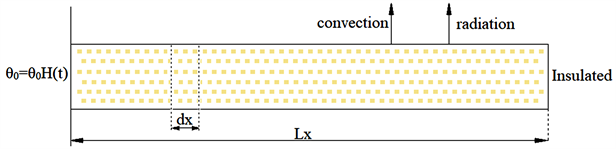Figure 1. Physical model

$k\frac{{\partial }^{2}T}{\partial {x}^{2}}=\rho {c}_{E}\frac{\partial T}{\partial t}+\tau \rho {c}_{E}\frac{{\partial }^{2}T}{\partial {t}^{2}}+\gamma {T}_{0}\frac{{\partial }^{2}u}{\partial t\partial x}+\tau \gamma {T}_{0}\frac{{\partial }^{3}u}{\partial {t}^{2}\partial x}+S+\tau {S}^{\prime }$ (1)

$\begin{array}{c}S=\frac{hP\left(1-\phi \right)}{A}\left(T-{T}_{0}\right)+\frac{\epsilon \sigma {F}_{f\text{-}a}P\left(1-\phi \right)}{A}\left({T}^{4}-{T}_{0}^{4}\right)+\phi \frac{{\rho }_{f}{c}_{E,f}�{\beta }_{f}KP}{{\nu }_{f}A}{\left(T-{T}_{0}\right)}^{2}\\ =\frac{{h}_{a}P\left(1-\phi \right)}{A}\frac{{\left(T-{T}_{0}\right)}^{m+1}}{{\left({T}_{b}-{T}_{0}\right)}^{m}}+\frac{\epsilon \sigma {F}_{f\text{-}a}P\left(1-\phi \right)}{A}\left({T}^{4}-{T}_{0}^{4}\right)+\phi \frac{{\rho }_{f}{c}_{E,f}�{\beta }_{f}KP}{{\nu }_{f}A}{\left(T-{T}_{0}\right)}^{2}\end{array}$ (2)

$\rho \frac{{\partial }^{2}u}{\partial {t}^{2}}=\left(\lambda +2\mu \right)\frac{{\partial }^{2}u}{\partial {x}^{2}}-\gamma \frac{\partial T}{\partial x}+{\mu }_{e}{H}_{0}^{2}\frac{{\partial }^{2}u}{\partial {x}^{2}}$ (3)

$\sigma =\left(\lambda +2\mu \right)\frac{\partial u}{\partial x}-\gamma T+{\mu }_{e}{H}_{0}^{2}\frac{\partial u}{\partial x}$ (4)

${x}^{\text{*}}={c}_{1}{\eta }_{1}x$${u}^{\text{*}}={c}_{1}{\eta }_{1}u$${t}^{\text{*}}={c}_{1}^{2}{\eta }_{1}t$${\tau }^{\text{*}}={c}_{1}^{2}{\eta }_{1}\tau$${\theta }^{*}=T/{T}_{0}$${\sigma }^{\text{*}}=\sigma /\mu$

$b=\frac{\gamma {T}_{0}}{\mu }$$g=\frac{\gamma }{\rho {c}_{E}}$$h=\frac{{\mu }_{e}{H}_{0}^{2}}{\mu }$${\beta }^{2}=\frac{\lambda +2\mu }{\mu }$$ϵ=\frac{{\mu }_{e}{H}_{0}^{2}}{\mu }$

${N}_{c}=\frac{k}{{\left(\rho {c}_{E}{c}_{1}\right)}^{2}}\frac{{h}_{a}P\left(1-\phi \right)}{A{\left({\theta }_{b}-{\theta }_{0}\right)}^{m}}$${N}_{r}=\frac{k\epsilon \sigma {F}_{f\text{-}a}P\left(1-\phi \right)}{{\left(\rho {c}_{E}{c}_{1}\right)}^{2}A}{T}_{0}^{3}$${N}_{p}=\frac{k}{{\left(\rho {c}_{E}{c}_{1}\right)}^{2}}\phi \frac{{\rho }_{f}{c}_{E,f}�{\beta }_{f}K{T}_{0}P}{{\nu }_{f}A}$。其中，c1为等温弹性波的传播速度， ${c}_{1}^{2}=\frac{\lambda +2\mu }{\rho }$${\eta }_{1}=\frac{\rho {c}_{E}}{k}$

$\frac{{\partial }^{2}\theta }{\partial {x}^{2}}=\left(1+\tau \frac{\partial }{\partial t}\right)\left(\frac{\partial \theta }{\partial t}+g\frac{{\partial }^{2}u}{\partial t\partial x}+{N}_{c}{\left(\theta -{\theta }_{0}\right)}^{m+1}+{N}_{r}\left({\theta }^{4}-{\theta }_{0}^{4}\right)+{N}_{p}{\left(\theta -{\theta }_{0}\right)}^{2}\right)$ (5)

${\beta }^{2}\frac{{\partial }^{2}u}{\partial {t}^{2}}=\left({\beta }^{2}+ϵ\right)\frac{{\partial }^{2}u}{\partial {x}^{2}}-b\frac{\partial \theta }{\partial x}$ (6)

$\sigma =\left({\beta }^{2}+ϵ\right)\frac{\partial u}{\partial x}-b\theta$ (7)

$\theta \left(x,t\right)=0,\text{\hspace{0.17em}}\text{\hspace{0.17em}}t=0$

$u\left(x,t\right)=0,\text{\hspace{0.17em}}\text{\hspace{0.17em}}t=0$

$\theta \left(x,t\right)={\theta }_{0}H\left(t\right),\text{\hspace{0.17em}}\text{\hspace{0.17em}}x=0$

$\sigma \left(x,t\right)=0,\text{\hspace{0.17em}}\text{\hspace{0.17em}}x=0$

$\frac{\partial \theta }{\partial x}=0,\text{\hspace{0.17em}}\text{\hspace{0.17em}}x=L$

$\sigma \left(x,t\right)=0,\text{\hspace{0.17em}}\text{\hspace{0.17em}}x=L$

3. 验证

$\lambda =\text{7}.\text{76}$$\mu =3.86×{10}^{10}$${\alpha }_{t}=1.78×{10}^{-5}$$С=383.1$$\rho =8954$${T}_{0}=293$

$\epsilon =0.161$${\beta }_{2}=4$$b=0.042$$g=1.61$${\theta }_{0}=1$${L}_{x}=1$$\tau =0.02$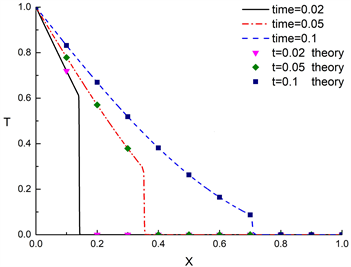(a) 温度分布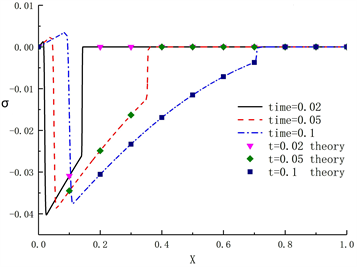(b) 应力分布

Figure 2. Model validation

4. 结果与讨论

4.1. 磁场强度对实心肋片温度和热应力的影响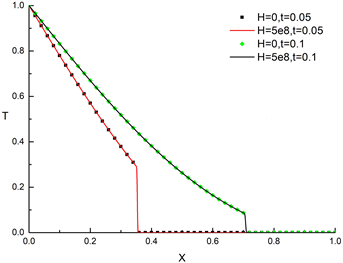(a) 电磁力对温度的影响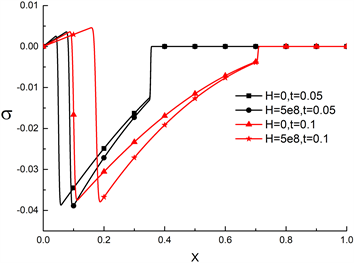(b) 电磁力对应力的影响

Figure 3. Influence of electromagnetic force on temperature and stress

4.2. 孔隙率对多孔肋片内温度和热应力的影响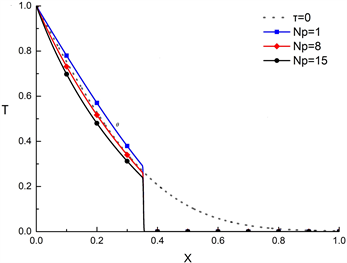(a) 温度分布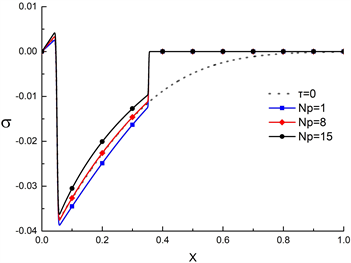(b) 应力分布

Figure 4. Temperature and stress distribution (Nr = 0, Nc = 0, t = 0.05)(a) 温度分布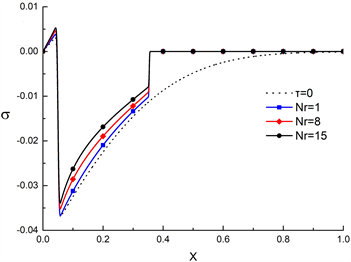(b) 应力分布

Figure 5. Temperature and stress distribution (Np = 8, Nc = 0, t = 0.05)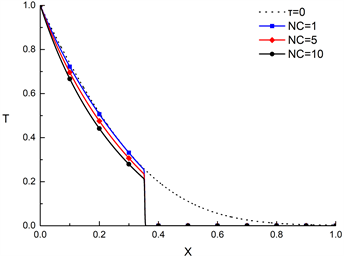(a) 温度分布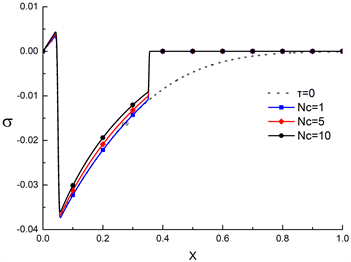(b) 应力分布

Figure 6. Temperature and stress distribution (Np = 8, Nr = 1, t = 0.05)

4.3. 周期性边界条件对多孔肋片内温度和热应力的影响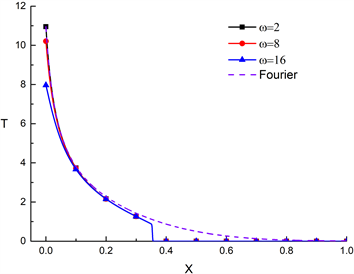(a) 温度分布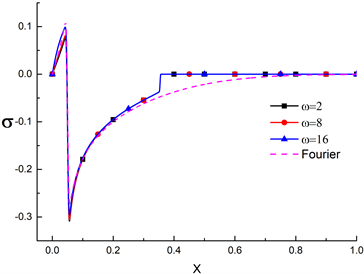(b) 应力分布

Figure 7. Temperature and stress distribution (Np = 8, Nr = 1, t = 0.05)

5. 结论

NOTES

*通讯作者。

  Kundu, B. and Lee, K.S. (2012) Fourier and Non-Fourier Heat Conduction Analysis in the Absorber Plates of a Flat-Plate Solar Collector. Solar Energy, 86, 3030-3039. https://doi.org/10.1016/j.solener.2012.07.011  Kundu, B. and Lee, K.S. (2013) A Non-Fourier Analysis for Transmitting Heat in Fins with Internal Heat Generation. International Journal of Heat and Mass Transfer, 64, 1153-1162. https://doi.org/10.1016/j.ijheatmasstransfer.2013.05.057  Kraus, A.D., Aziz, A., Welty, J. and Sekulic, D.P. (2001) Extended Surface Heat Transfer. Applied Mechanics Reviews, 54, B92. https://doi.org/10.1115/1.1399680  Kiwan, S. and Al Nimr, M.A. (2001) Using Porous Fins for Heat Transfer Enhancement. Journal of Heat Transfer, 123, 790-795. https://doi.org/10.1115/1.1371922  Hoshyar, H.A., Ganji, D.D. and Abbasi, M. (2015) Analytical Solution for Porous Fin with Temperature-Dependent Heat Generation via Homotopy Perturbation Method. International Journal of Advances in Applied Mathematics and Mechanics, 2, 15-22.  Darvishi, M.T., Gorla, R.S.R., Khani, F., et al. (2016) Thermal Analysis of Natural Convection and Radiation in a Fully Wet Porous Fin. International Journal of Numerical Methods for Heat and Fluid Flow, 26, 2419-2431. https://doi.org/10.1108/HFF-06-2015-0230  Gorla, R.S.R. and Bakier, A.Y. (2011) Thermal Analysis of Natural Convection and Radiation in Porous Fins. International Communincations in Heat and Mass Transfer, 38, 638-645. https://doi.org/10.1016/j.icheatmasstransfer.2010.12.024  Hatami, M. and Ganji, D.D. (2014) Thermal Behavior of Longitudinal Convective-Radiative Porous Fins with Different Section Shapes and Ceramic Materials (SiC and Si3N4). Ceramics International, 40, 6765-6775. https://doi.org/10.1016/j.ceramint.2013.11.140  Atouei, S.A., Hosseinzadeh, K.H., Hatami, M., et al. (2015) Heat Transfer Study on Convective-Radiative Semispherical Fins with Temperature-Dependent Properties and Heat Generation Using Efficient Computational Methods. Applied Thermal Engineering, 89, 299-305. https://doi.org/10.1016/j.applthermaleng.2015.05.084  El-Maghraby, N.M. and Yossef, H.M. (2003) State Space Approach to Generalized Thermoelastic Problem with Thermomechanical Shock. Applied Mathematics and Computation, 156, 577-586. https://doi.org/10.1016/j.amc.2003.08.009  He, T.H., Shen, Y.P. and Tian, X.G. (2003) A Two-Dimensional Generalized Thermal Shock Problem for a Half-Space in Electromagneto-Thermoelasticity. In-ternational Journal of Engineering Science, 42, 809-823. https://doi.org/10.1016/j.ijengsci.2003.09.006  杨世铭, 陶文铨. 传热学[M]. 第四版. 北京: 高等教育出版社, 2006.  吴吉春. 地下水动力学[M]. 北京: 中国水利水电出版社, 2009.  Mehraban, M., Khosra-vi-Nikou, M.R. and Shaahmadi, F. (2019) Thermal Behaviour of Convective-Radiative Porous Fins under Periodic Thermal Conditions. The Canadian Journal of Chemical Engineering, 97, 821-828. https://doi.org/10.1002/cjce.23240